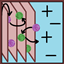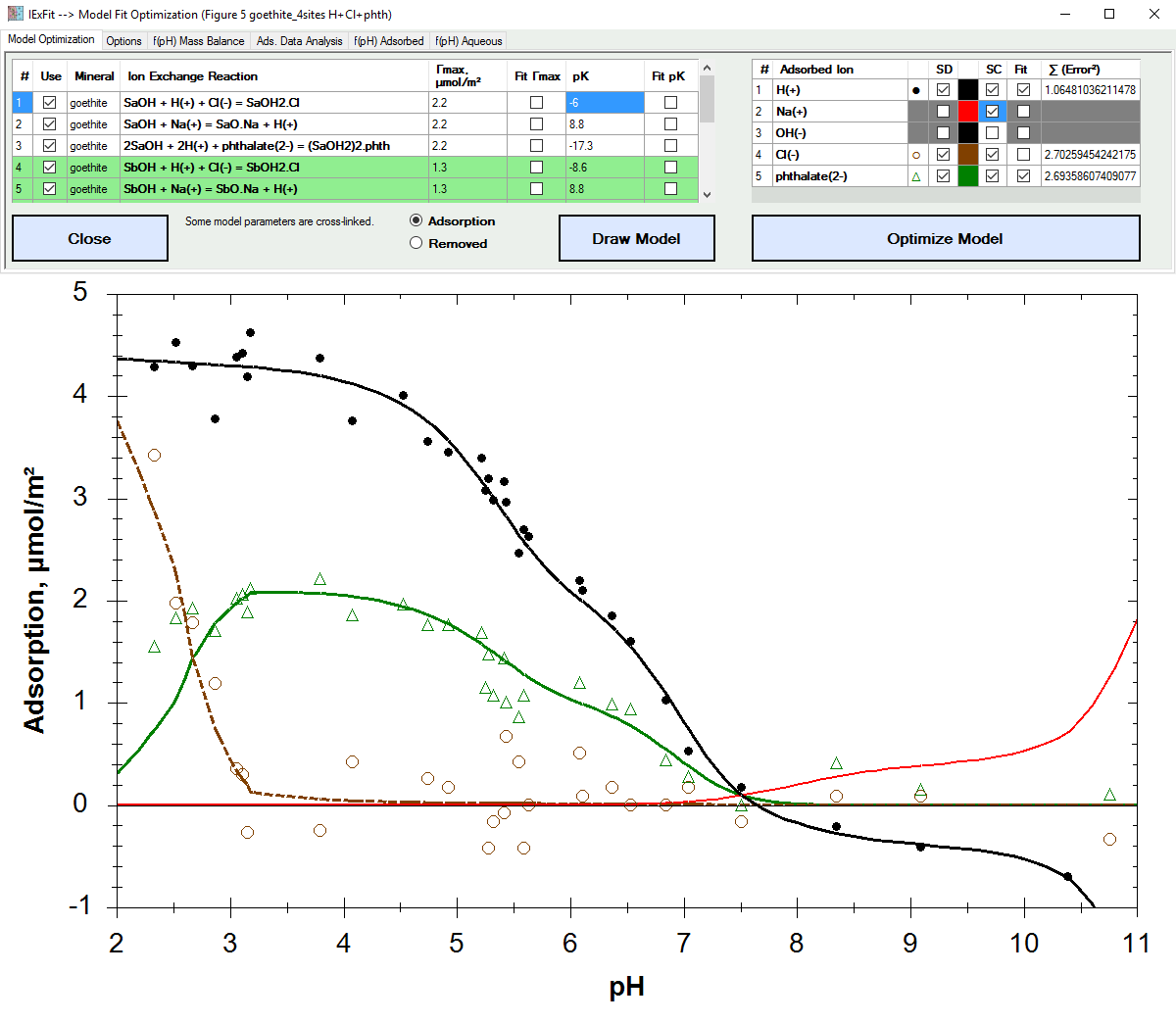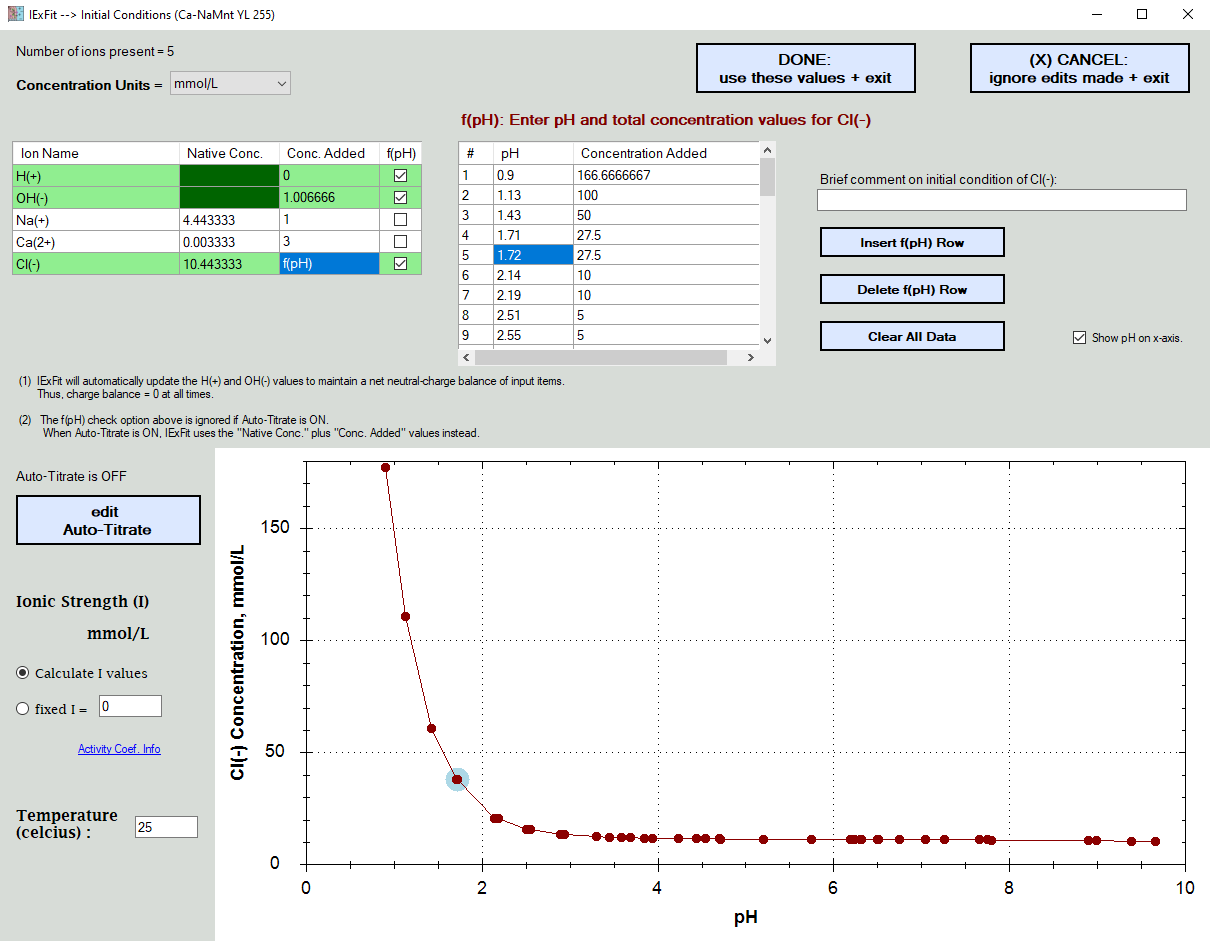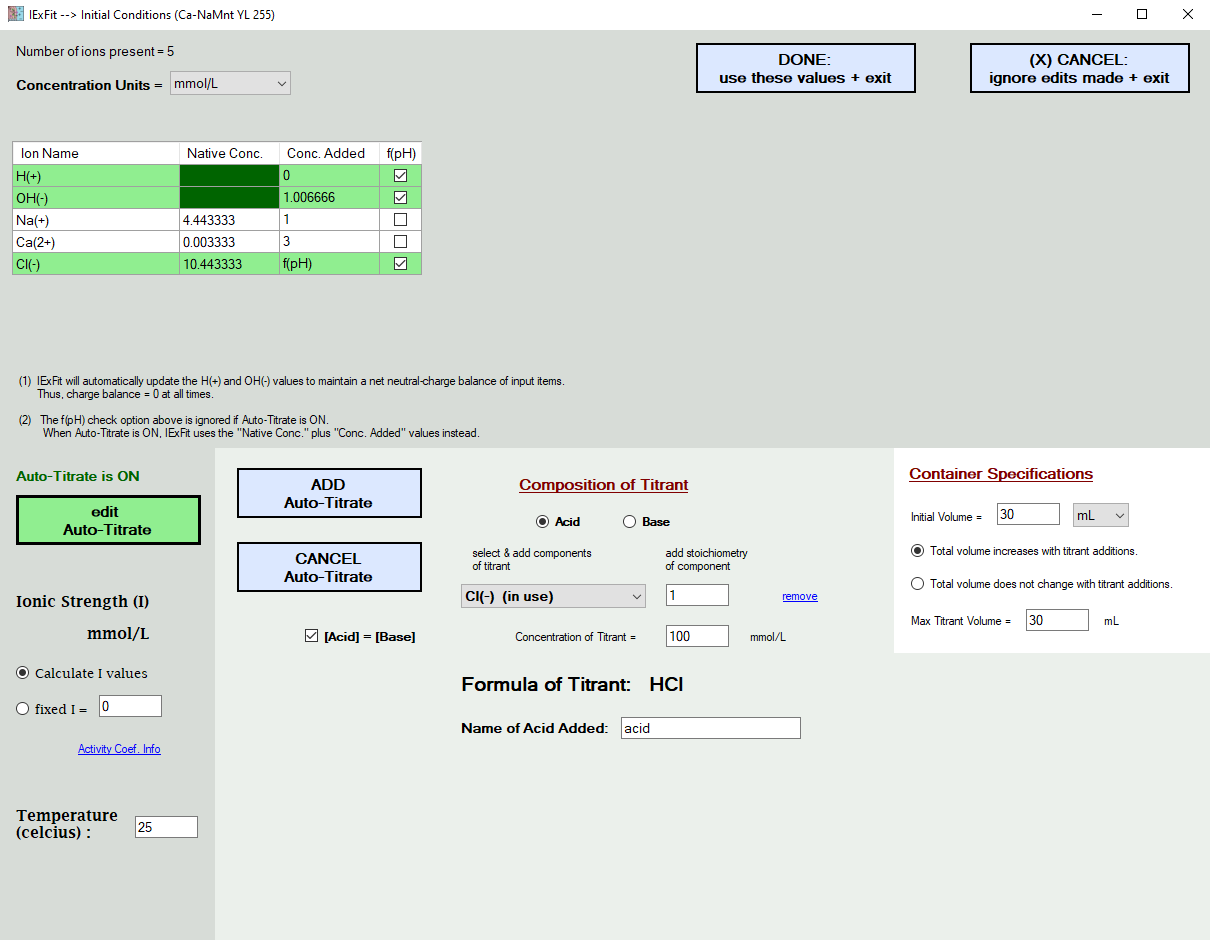Alfisol, Item 007: IExFit, version 3.4 Fit adsorption data based on ion exchange reaction models. Display solid & liquid & gas phase speciation diagrams as a funciton of pH & redox conditions.# Software Overview## Step 2: Enter Initial Conditions

Here you enter the concentration of the ions present in your experiments.

In the example shown below,
.... H(+) and OH(-) values are set automatically for electroneutrality based on what was already added.
.... the total Na(+) and Ca(2+) concentrations added were constant
.... the Cl(-) concentrations added were pH dependent due to the variable addition of HCl to control the pH

In the example shown below, the Cl(-) ions were also later determined to exist in the solid surface and thus contribute to the total Cl(-) ion concentrations present. Rather than having to re-enter all the pH dependent values, you simply edit the "Native Concentration" cellbox information.

In the picture further below, the addition of H(+) and Cl(-) ions are simulated in the form of HCl titrant additions. When you choose to do this, the f(pH) additions in the grid above are ignored. After all, you cannot add Cl(-) as a function of pH and also add it with the acid titrant at the same time. You either tell IExFit the actual acid values used, or you ask IExFit to simulate it. It will not let you do both at the same time. You also enter here the temperature of the experiment. This will temperature-correct the database equilibrium constants.

You also enter the ionic strength condition, which is either at a fixed value or is calculated by IExFit based on the ions remaining in solution. IExFit uses the Davies Equation to calculate the activity coefficient of each ion in the aqueous phase, which is then used to correlate ion activity with ion concentration.

Example 1:Example 2: